This Week at Northside - Sunday, November 15

# Upcoming Important Dates

Tuesday, November 17 - LSC meeting/Friends of Northside meeting (online)
Thursday, November 19 - Online Parent/Teacher Conferences/No School/LSC elections/FON Senior Parent College Night
Wednesday, November 25-Friday, November 27 - No School - Thanksgiving break
Thursday, December 3 - Parent Virtual Trivia Night

Dates for NCP, ISAC, University of Michigan, and Illinois State's financial aid workshops and presentations are listed below in the financial aid article.

 table div table+table+table+table div table{width:100%;padding:0}table div table+table+table+table div table img{width:96.23%;padding:0;float:none}table div table+table+table+table div table td{width:100%;padding:0 1.88% 18px}/* styles */▪ The 2020-2021 Annual Appeal Now Has PayPal to Make Donations
▪ LSC Meeting on Tuesday, November 17
▪ Friends of Northside Google Meet on November 17
▪ No School This Thursday, November 19: Sign-Up for Online Parent-Teacher Conferences
▪ LSC Elections on November 19
▪ Our Senior Parent College Night is Thursday, November 19
▪ Thank You for Your Help With our Online Open House
▪ Winter Sports Open for Registration
▪ AP Exam and Payment Information
▪ School CNXT: Parent-NCP Communication
▪ College Application and Essay Resources
▪ College Representative Visits
▪ Verto Education Virtual College Fair
▪ Upcoming Virtual Financial Aid Presentations
▪ Photo Submissions Requested for NCP Stampede!
▪ Ways for Parents to Stay in Touch
 ▪ The 2020-2021 Annual Appeal Now Has PayPal to Make Donations
 ▪ LSC Meeting on Tuesday, November 17
 ▪ Friends of Northside Google Meet on November 17
 ▪ No School This Thursday, November 19: Sign-Up for Online Parent-Teacher Conferences
 ▪ LSC Elections on November 19
 ▪ Our Senior Parent College Night is Thursday, November 19
 ▪ Thank You for Your Help With our Online Open House
 ▪ Winter Sports Open for Registration
 ▪ AP Exam and Payment Information
 ▪ School CNXT: Parent-NCP Communication
 ▪ College Application and Essay Resources
 ▪ College Representative Visits
 ▪ Verto Education Virtual College Fair
 ▪ Upcoming Virtual Financial Aid Presentations
 ▪ Photo Submissions Requested for NCP Stampede!
 ▪ Ways for Parents to Stay in Touch
 table div table+table+table+table+table+table div table{width:100%;padding:0}table div table+table+table+table+table+table div table img{width:96.23%;padding:0;float:none}table div table+table+table+table+table+table div table td{width:100%;padding:0 1.88% 18px}/* styles */# The 2020-2021 Annual Appeal Now Has PayPal to Make Donations

Dear Parents,

In a typical school year, many parents make donations to the Annual Appeal campaign at a table set-up by Friends of Northside during report card pick-up and teacher conferences.

This year, Friends of Northside has set-up processing with PayPal if you would like to donate online directly from your checking or savings account. PayPal is a convenient way to transfer funds and support Northside.

As noted previously, our goal for this campaign is to raise \$500,000. A donation of \$10, \$100, \$1,000, \$10,000, or whatever you can afford can help bridge the gap between what CPS provides and what Northside needs to continue to be the great school it is.

Want to donate now? Click HERE. All donations are tax-deductible, and any amount is greatly appreciated!

Questions? Email info@friendsofnorthside.org to contact Friends of Northside.

Want to donate now? Click HERE. All donations are tax-deductible, and any amount is greatly appreciated!

 table div table+table+table+table+table+table+table+table+table div table{width:100%;padding:0}table div table+table+table+table+table+table+table+table+table div table img{width:96.23%;padding:0;float:none}table div table+table+table+table+table+table+table+table+table div table td{width:100%;padding:0 1.88% 18px}/* styles */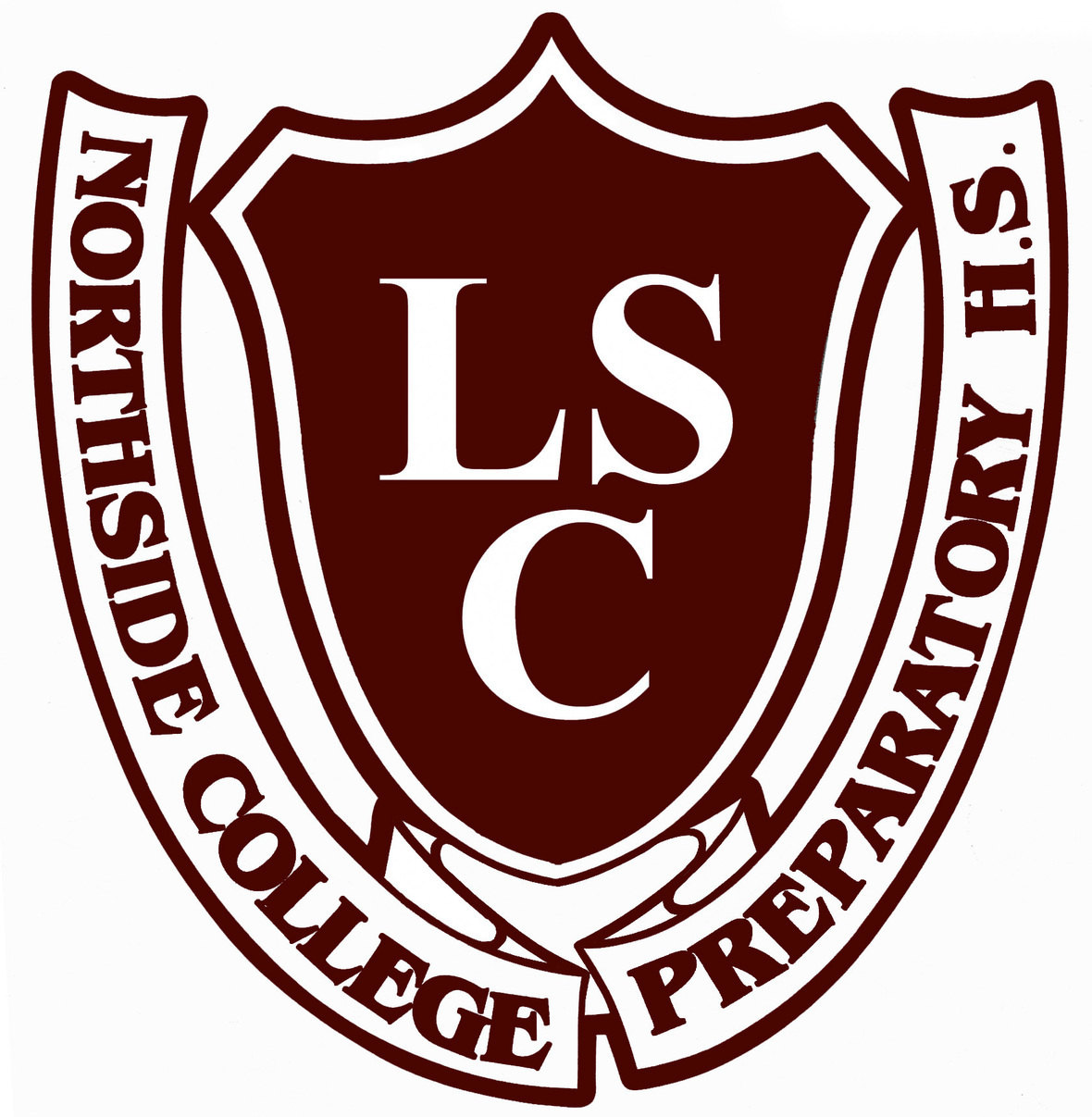# LSC Meeting

Tuesday, November 17
6:30 pm
Zoom Meet

The agenda for our monthly meeting can be found HERE.

Meeting ID: 878 3779 8658
Passcode: 680330
One tap mobile
+13126266799,,87837798658# US (Chicago)

 table div table+table+table+table+table+table+table+table+table+table+table div table{width:100%;padding:0}table div table+table+table+table+table+table+table+table+table+table+table div table img{width:96.23%;padding:0;float:none}table div table+table+table+table+table+table+table+table+table+table+table div table td{width:100%;padding:0 1.88% 18px}/* styles */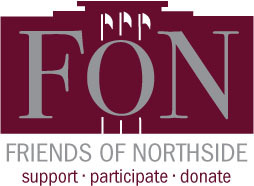# Friends of Northside

Tuesday, November 17
7:00 pm

Please join Friends of Northside for their upcoming meeting.

Friends of Northside (FON) is the “one-stop-shop” for NCP families to get involved. FON facilitates communication between parents and the school, organizes parent volunteers, plans and sponsors social/educational events, and raises much-needed funds to bridge the gap due to CPS budget cuts. FON supports the school’s mission to maintain and enhance the learning experience for all students of Northside College Prep High School.

To find out more about Friends of Northside, please visit their website HERE.

 table div table+table+table+table+table+table+table+table+table+table+table+table+table div table{width:100%;padding:0}table div table+table+table+table+table+table+table+table+table+table+table+table+table div table img{width:96.23%;padding:0;float:none}table div table+table+table+table+table+table+table+table+table+table+table+table+table div table td{width:100%;padding:0 1.88% 18px}/* styles */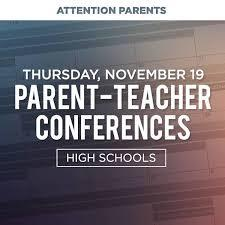# No School This Thursday: Sign-Up for Online Parent-Teacher Conferences

Thursday, November 19

This Thursday, November 19, Northside Prep will host virtual Parent/Teacher conferences from 12:00 pm - 6:00 pm.

To register for conferences, please do the following:
1. Determine which teachers you need to meet with. This is a great time to talk with your student about their progress and their work during the first quarter.
2. Using this spreadsheet, locate your student’s teachers, and click on the link to schedule your virtual conference. This will take you to the teachers’ individual conference calendar.
3. Confirm your conference appointments. After you register, you will receive a confirmation email for each appointment that will also include the Google Meet link for your conferences. There will be a different Meet Code for every conference that you schedule.

Conferences will be five minutes long. We ask that you are on time for your conference and recognize that teachers will have to leave your conference to join another immediately after and may have to end the conference quickly. We recommend that you do not sign up for back to back conferences so that you have time between to reset and find the link for your next conference.

This year, we are asking that you only sign up for conferences where it is necessary that you speak with that teacher. It is important to have conference slots available for parents of students who are struggling or in need of additional support.

As a reminder, if you are unable to schedule a conference with a teacher, they are always available via email. All teacher email addresses can be found on our website.

 table div table+table+table+table+table+table+table+table+table+table+table+table+table+table+table div table{width:100%;padding:0}table div table+table+table+table+table+table+table+table+table+table+table+table+table+table+table div table img{width:96.23%;padding:0;float:none}table div table+table+table+table+table+table+table+table+table+table+table+table+table+table+table div table td{width:100%;padding:0 1.88% 18px}/* styles */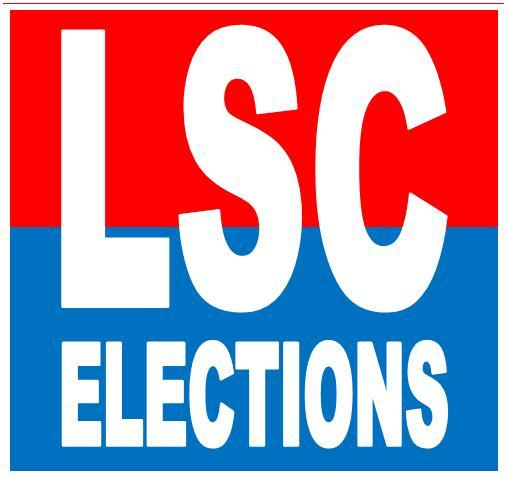# LSC Elections on November 19

High School LSC elections will be held on November 19 between 7:00 am and 7:00 pm.

Due to COVID-19, parents and guardians can choose between either voting in-person at their respective schools, submitting their ballots by mail, or dropping off their mail-in ballots. For in-person voting, the district is implementing stringent health and safety measures, including social distancing, face covering requirements, and completing a health screener and temperature check prior to entering school buildings. The district will also offer curbside voting for individuals with disabilities.

If you have questions about voting in LSC Elections, contact the CPS Office of LSC Relations at lsc@cps.edu or 773-553-1400 or visit their website HERE.

 table div table+table+table+table+table+table+table+table+table+table+table+table+table+table+table+table+table div table{width:100%;padding:0}table div table+table+table+table+table+table+table+table+table+table+table+table+table+table+table+table+table div table img{width:96.23%;padding:0;float:none}table div table+table+table+table+table+table+table+table+table+table+table+table+table+table+table+table+table div table td{width:100%;padding:0 1.88% 18px}/* styles */# FON Senior Parent College Night (Virtual)

Thursday, November 19
7:00 pm
Zoom Meeting

This is your opportunity to hear from the parents of recent Northside graduates about what transitioning from high school to college has been like during the pandemic. The pandemic has affected all students’ college preparation but each class faces distinct challenges relating to where they are in that process. Although parents of students from all grades are welcome to attend, the panelists will be focusing on topics of most immediate interest to parents of seniors. We hope to schedule another Parent College Night in the spring in conjunction with the Counseling Department that will be more all-encompassing in scope.

## Join Zoom Meeting

https://us04web.zoom.us/j/74821885251?pwd=UHIwRlhrUXNTMjhPeERTNjZkOGxKUT09

Meeting ID: 748 2188 5251
Passcode: 3YrDuQ

 table div table+table+table+table+table+table+table+table+table+table+table+table+table+table+table+table+table+table+table+table div table{width:100%;padding:0}table div table+table+table+table+table+table+table+table+table+table+table+table+table+table+table+table+table+table+table+table div table img{width:96.23%;padding:0;float:none}table div table+table+table+table+table+table+table+table+table+table+table+table+table+table+table+table+table+table+table+table div table td{width:100%;padding:0 1.88% 18px}/* styles */# Parent Virtual Trivia Night

Thursday, December 3
7:30 pm
Zoom Meeting

All are welcome to attend this online event! Grab a snack and join in the fun online. No trivia knowledge needed.

A Zoom link will be shared closer to the event.

 table div table+table+table+table+table+table+table+table+table+table+table+table+table+table+table+table+table+table+table+table+table+table div table{width:100%;padding:0}table div table+table+table+table+table+table+table+table+table+table+table+table+table+table+table+table+table+table+table+table+table+table div table img{width:96.23%;padding:0;float:none}table div table+table+table+table+table+table+table+table+table+table+table+table+table+table+table+table+table+table+table+table+table+table div table td{width:100%;padding:0 1.88% 18px}/* styles */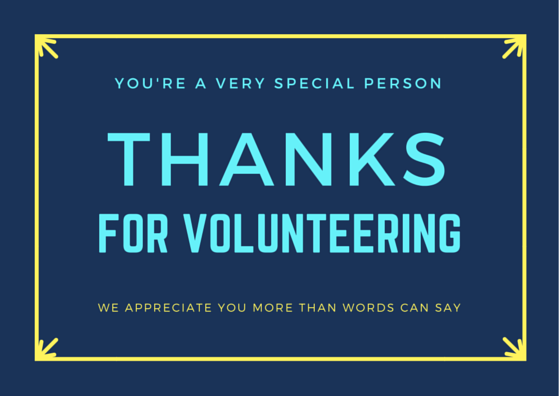# Thank You for Your Help with the Open House!

We are so grateful for the many parents and students sent in photos, stories, and videos to personalize our virtual Open House videos!

Today's event was successful only because of our amazing and supportive community. We couldn't have done it without you!

 table div table+table+table+table+table+table+table+table+table+table+table+table+table+table+table+table+table+table+table+table+table+table+table+table+table div table{width:100%;padding:0}table div table+table+table+table+table+table+table+table+table+table+table+table+table+table+table+table+table+table+table+table+table+table+table+table+table div table img{width:96.23%;padding:0;float:none}table div table+table+table+table+table+table+table+table+table+table+table+table+table+table+table+table+table+table+table+table+table+table+table+table+table div table td{width:100%;padding:0 1.88% 18px}/* styles */# Winter Sports Are Now Open for Registration

All winter student-athletes must register to participate in a winter sport. Visit the NCP athletics website HERE and click on the Activity Registration tab. In addition to registering, students must have a current physical on file before they try out or practice. Contact athletic director Henry Henderson at hhenderson@cps.edu for more information.

## 2020 - 2021 Northside College Prep winter sports information

Boys & Girls basketball along with wrestling has been postponed until later in the school year

Boys & Girls Bowling
Tryout/practice will take place at Habetler Bowl (5250 N. Northwest Hwy) Thursday, November 19 from 4:00 pm - 6:00 pm. Contact coaches for more information:

▪ Girls Bowling - Kia Giddings at klgiddings@cps.edu
▪ Boys Bowling - Dave Kresl at dkresl@cps.edu
 ▪ Girls Bowling - Kia Giddings at klgiddings@cps.edu
 ▪ Boys Bowling - Dave Kresl at dkresl@cps.edu

Boys Swimming
Tryouts/practice will take place in the Northside Aquatics Center on Monday, November 16 from 4:30 pm to 7:00 pm. Contact head coach Natalie O'Connell at noconnell1@cps.edu for more information.

Cheer
Tryouts will take place on Thursday, November 19 and Friday, November 20 from 4:30 pm to 6:30 pm in the NCP Auditorium. Contact head coach Kamarie Bostic at kjbostic@cps.edu for more information.

Poms
Regular practices will be in the gym on Tuesdays and Thursdays weekly from 4:00 pm to 5:30 pm. Contact head coach Ellie Duerst at erduerst@cps.edu for more information.

## New COVID Daily Health Screening

All Northside student-athletes must complete the CPS Daily Health Screener before attending a game or practice.

 table div table+table+table+table+table+table+table+table+table+table+table+table+table+table+table+table+table+table+table+table+table+table+table+table+table+table+table div table{width:100%;padding:0}table div table+table+table+table+table+table+table+table+table+table+table+table+table+table+table+table+table+table+table+table+table+table+table+table+table+table+table div table img{width:96.23%;padding:0;float:none}table div table+table+table+table+table+table+table+table+table+table+table+table+table+table+table+table+table+table+table+table+table+table+table+table+table+table+table div table td{width:100%;padding:0 1.88% 18px}/* styles */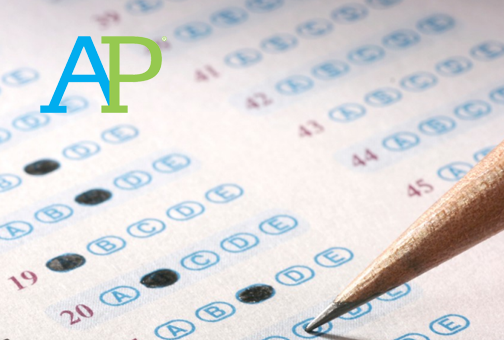# AP Exam and Payment Information

AP Exams will take place May 3-7 and May 10-14. AP Exam fee payments will be due by Friday, December 4 at 3:00 pm.

There is currently a technical issue with the collection of AP Exam fees, which are completely separate from your school fees. Your continued patience is very much appreciated. An announcement will be made to all students, teachers, and families to ensure that you are able to pay on time.

For reference, the AP exam cost is \$110 per exam. If you completed your family income form and have been approved, the exam cost is \$15 per exam. For students enrolled in AP Seminar and Research, the exam cost is \$143.

 table div table+table+table+table+table+table+table+table+table+table+table+table+table+table+table+table+table+table+table+table+table+table+table+table+table+table+table+table+table+table div table{width:100%;padding:0}table div table+table+table+table+table+table+table+table+table+table+table+table+table+table+table+table+table+table+table+table+table+table+table+table+table+table+table+table+table+table div table img{width:96.23%;padding:0;float:none}table div table+table+table+table+table+table+table+table+table+table+table+table+table+table+table+table+table+table+table+table+table+table+table+table+table+table+table+table+table+table div table td{width:100%;padding:0 1.88% 18px}/* styles */# All NCP Communications Now Being Sent Via CNXT

NCP is now using SchoolCNXT to communicate important information to parents and the school community. All families should have received a text message and/or email communication about signing up.

If you did not receive that communication or need access to your student's account, you can gain access by using this tool on Northside's website.

 table div table+table+table+table+table+table+table+table+table+table+table+table+table+table+table+table+table+table+table+table+table+table+table+table+table+table+table+table+table+table+table+table div table{width:100%;padding:0}table div table+table+table+table+table+table+table+table+table+table+table+table+table+table+table+table+table+table+table+table+table+table+table+table+table+table+table+table+table+table+table+table div table img{width:96.23%;padding:0;float:none}table div table+table+table+table+table+table+table+table+table+table+table+table+table+table+table+table+table+table+table+table+table+table+table+table+table+table+table+table+table+table+table+table div table td{width:100%;padding:0 1.88% 18px}/* styles */# College Application and Essay Resources

Hello, Class of 2021 Parents,

Time is moving at light speed and the November college application deadlines are quickly approaching. New York University has you covered with helpful tips and best practices as your students prepare to hit submit on their college applications!

Here are a few articles that will assist you in supporting your students as they tackle their college applications and essays.

If you have any questions or concerns, do not hesitate to contact Ms. Moore at mcmoore6@cps.edu or email your student’s respective school counselor. We are here to support your family with navigating the next steps in the college admissions process.

▪ 3 Tips to Choose the Right Common App Essay Prompt (click here)
▪ What I Wish I Knew Before Applying to College (click here)

 table div table+table+table+table+table+table+table+table+table+table+table+table+table+table+table+table+table+table+table+table+table+table+table+table+table+table+table+table+table+table+table+table+table+table+table div table{width:100%;padding:0}table div table+table+table+table+table+table+table+table+table+table+table+table+table+table+table+table+table+table+table+table+table+table+table+table+table+table+table+table+table+table+table+table+table+table+table div table img{width:96.23%;padding:0;float:none}table div table+table+table+table+table+table+table+table+table+table+table+table+table+table+table+table+table+table+table+table+table+table+table+table+table+table+table+table+table+table+table+table+table+table+table div table td{width:100%;padding:0 1.88% 18px}/* styles */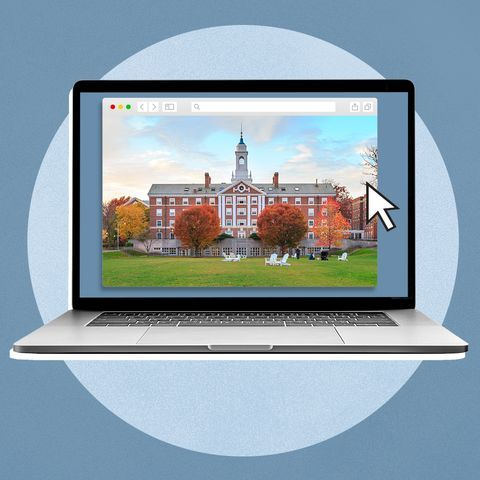# College Representative Visits

The NCP Counseling Department is offering virtual postsecondary visits for Northside Prep students and parents. We are offering virtual postsecondary visits to ensure all families have the opportunity to explore various postsecondary pathways, engage with the postsecondary representatives, and obtain answers to pertinent questions or concerns. Virtual postsecondary visits are offered after school from 3:15-4:00 pm, and during Flex Blocks 1-4 on Wednesdays.

To date, college, military, and GAP Year representatives are scheduled to connect with NCP students virtually and the list is growing every day. Click HERE to view the list of upcoming and past postsecondary visits. Also, check out the messages from the admissions representatives and the additional resources they shared during their visits.

*Please note, all virtual visits will be conducted via CPS Google Meets and no email addresses outside CPS will be permitted into the virtual postsecondary visits. To protect the privacy and safety of students, parents will only be permitted to access virtual visits if their respective student is present and the student will be required to log in via their CPS Gmail account. All postsecondary visits will be managed and monitored by an NCP staff member.

## Verto Education Virtual Open House and College Fair

Verto Education provides an affordable and innovative way for students to incorporate travel into their four-year college experience. Spending a few months abroad before diving into the typical college grind allows students to show up on campus rejuvenated with a better understanding of themselves and what they want to do with their lives.

With Verto Education, you know what the future holds. Verto students become global citizens and start college by finding clarity, meaning, and purpose that will guide them to become the leaders our world needs. Get the answers to all of your questions about Verto during a Virtual Open House.

College Fair
Tuesday, November 17, 1:00 pm - 4:00 pm
Register HERE

Verto is extremely proud to partner with a quickly growing consortium of forward-thinking colleges and universities — enabling students to see the world, gain academic knowledge, and meaningful life experience while receiving college credit.

Change the World International Honors Program: Two semesters, a year of international immersion, full-time credits for honors-level academics, and one transformative capstone project. Tuition is offered on a sliding scale and is based on household income.

Verto Opportunity Grant: For qualifying families, students can receive up to \$10,000 in grant funding to attend a Verto semester in Latin America or Hawaii.

 table div table+table+table+table+table+table+table+table+table+table+table+table+table+table+table+table+table+table+table+table+table+table+table+table+table+table+table+table+table+table+table+table+table+table+table+table+table+table+table div table{width:100%;padding:0}table div table+table+table+table+table+table+table+table+table+table+table+table+table+table+table+table+table+table+table+table+table+table+table+table+table+table+table+table+table+table+table+table+table+table+table+table+table+table+table div table img{width:96.23%;padding:0;float:none}table div table+table+table+table+table+table+table+table+table+table+table+table+table+table+table+table+table+table+table+table+table+table+table+table+table+table+table+table+table+table+table+table+table+table+table+table+table+table+table div table td{width:100%;padding:0 1.88% 18px}/* styles */# Upcoming Virtual Financial Aid Presentations

▪ Tuesday, November 17, 6:00-8:00 pm CT

▪ Wednesday, November 18, 4:00-5:00 pm
▪ Thursday, November 19, 6:30-7:30 pm

Presentations

Net Price Calculators

## Update: Recursos de presentación en Español

▪ Solicitud Alternativa para aplicar para la Subvención MAP de Illinois 2020-2021 (click here)
 ▪ Solicitud Alternativa para aplicar para la Subvención MAP de Illinois 2020-2021 (click here)
 table div table+table+table+table+table+table+table+table+table+table+table+table+table+table+table+table+table+table+table+table+table+table+table+table+table+table+table+table+table+table+table+table+table+table+table+table+table+table+table+table+table+table+table div table{width:100%;padding:0}table div table+table+table+table+table+table+table+table+table+table+table+table+table+table+table+table+table+table+table+table+table+table+table+table+table+table+table+table+table+table+table+table+table+table+table+table+table+table+table+table+table+table+table div table img{width:96.23%;padding:0;float:none}table div table+table+table+table+table+table+table+table+table+table+table+table+table+table+table+table+table+table+table+table+table+table+table+table+table+table+table+table+table+table+table+table+table+table+table+table+table+table+table+table+table+table+table div table td{width:100%;padding:0 1.88% 18px}/* styles */# CPS Scholarship Notifications and College Events Around the City

For more details about upcoming events and to access the most recent updates, (click HERE) to view the College Calendar on the Northside Prep Counseling Website. Also, for additional scholarship opportunities visit the Northside College Prep Counseling page (click HERE).

Tuesday, November 17
1:00 pm - Verto Education Virtual College Fair

Tuesday, December 1
App Closes: Act Six Leadership Scholarship

Sunday, December 6
App Closes: Hispanic Heritage Foundation’s Youth Awards

 table div table+table+table+table+table+table+table+table+table+table+table+table+table+table+table+table+table+table+table+table+table+table+table+table+table+table+table+table+table+table+table+table+table+table+table+table+table+table+table+table+table+table+table+table+table div table{width:100%;padding:0}table div table+table+table+table+table+table+table+table+table+table+table+table+table+table+table+table+table+table+table+table+table+table+table+table+table+table+table+table+table+table+table+table+table+table+table+table+table+table+table+table+table+table+table+table+table div table img{width:96.23%;padding:0;float:none}table div table+table+table+table+table+table+table+table+table+table+table+table+table+table+table+table+table+table+table+table+table+table+table+table+table+table+table+table+table+table+table+table+table+table+table+table+table+table+table+table+table+table+table+table+table div table td{width:100%;padding:0 1.88% 18px}/* styles */# Photo Submissions Requested for NCP Stampede!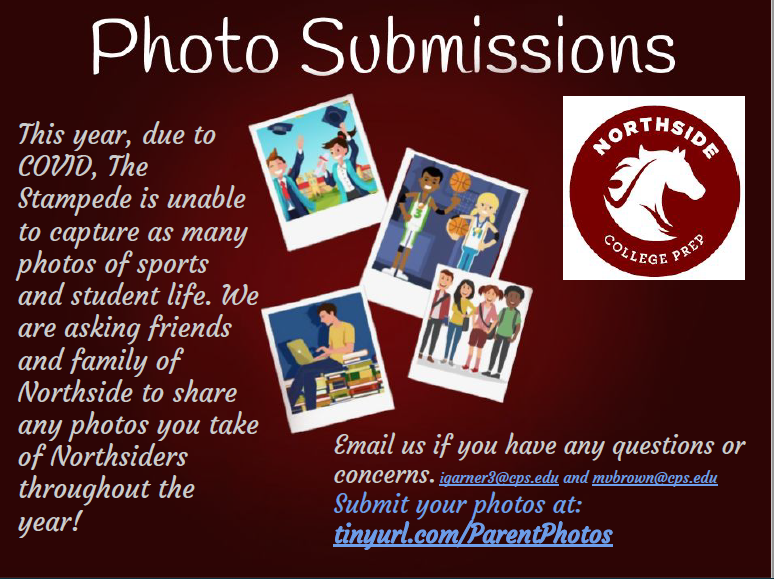table div table+table+table+table+table+table+table+table+table+table+table+table+table+table+table+table+table+table+table+table+table+table+table+table+table+table+table+table+table+table+table+table+table+table+table+table+table+table+table+table+table+table+table+table+table+table+table+table div table{width:100%;padding:0}table div table+table+table+table+table+table+table+table+table+table+table+table+table+table+table+table+table+table+table+table+table+table+table+table+table+table+table+table+table+table+table+table+table+table+table+table+table+table+table+table+table+table+table+table+table+table+table+table div table img{width:96.23%;padding:0;float:none}table div table+table+table+table+table+table+table+table+table+table+table+table+table+table+table+table+table+table+table+table+table+table+table+table+table+table+table+table+table+table+table+table+table+table+table+table+table+table+table+table+table+table+table+table+table+table+table+table div table td{width:100%;padding:0 1.88% 18px}/* styles */# Do you have news or team results to share with the Northside parent community?

 table div table+table+table+table+table+table+table+table+table+table+table+table+table+table+table+table+table+table+table+table+table+table+table+table+table+table+table+table+table+table+table+table+table+table+table+table+table+table+table+table+table+table+table+table+table+table+table+table+table+table+table div table{width:100%;padding:0}table div table+table+table+table+table+table+table+table+table+table+table+table+table+table+table+table+table+table+table+table+table+table+table+table+table+table+table+table+table+table+table+table+table+table+table+table+table+table+table+table+table+table+table+table+table+table+table+table+table+table+table div table img{width:96.23%;padding:0;float:none}table div table+table+table+table+table+table+table+table+table+table+table+table+table+table+table+table+table+table+table+table+table+table+table+table+table+table+table+table+table+table+table+table+table+table+table+table+table+table+table+table+table+table+table+table+table+table+table+table+table+table+table div table td{width:100%;padding:0 1.88% 18px}/* styles */# Support Northside - Shop AmazonSmile

AmazonSmile is a simple and automatic way to support Northside every time you shop.

Please note that our AmazonSmile name is Friends of "North" "Side" (North Side - two words at AmazonSmile).

 table div table+table+table+table+table+table+table+table+table+table+table+table+table+table+table+table+table+table+table+table+table+table+table+table+table+table+table+table+table+table+table+table+table+table+table+table+table+table+table+table+table+table+table+table+table+table+table+table+table+table+table+table+table div table{width:100%;padding:0}table div table+table+table+table+table+table+table+table+table+table+table+table+table+table+table+table+table+table+table+table+table+table+table+table+table+table+table+table+table+table+table+table+table+table+table+table+table+table+table+table+table+table+table+table+table+table+table+table+table+table+table+table+table div table img{width:96.23%;padding:0;float:none}table div table+table+table+table+table+table+table+table+table+table+table+table+table+table+table+table+table+table+table+table+table+table+table+table+table+table+table+table+table+table+table+table+table+table+table+table+table+table+table+table+table+table+table+table+table+table+table+table+table+table+table+table+table div table td{width:100%;padding:0 1.88% 18px}/* styles */# Follow Us on Social Media

 table div table+table+table+table+table+table+table+table+table+table+table+table+table+table+table+table+table+table+table+table+table+table+table+table+table+table+table+table+table+table+table+table+table+table+table+table+table+table+table+table+table+table+table+table+table+table+table+table+table+table+table+table+table+table+table div table{width:100%;padding:0}table div table+table+table+table+table+table+table+table+table+table+table+table+table+table+table+table+table+table+table+table+table+table+table+table+table+table+table+table+table+table+table+table+table+table+table+table+table+table+table+table+table+table+table+table+table+table+table+table+table+table+table+table+table+table+table div table img{width:96.23%;padding:0;float:none}table div table+table+table+table+table+table+table+table+table+table+table+table+table+table+table+table+table+table+table+table+table+table+table+table+table+table+table+table+table+table+table+table+table+table+table+table+table+table+table+table+table+table+table+table+table+table+table+table+table+table+table+table+table+table+table div table td{width:100%;padding:0 1.88% 18px}/* styles */table div table+table+table+table+table+table+table+table+table+table+table+table+table+table+table+table+table+table+table+table+table+table+table+table+table+table+table+table+table+table+table+table+table+table+table+table+table+table+table+table+table+table+table+table+table+table+table+table+table+table+table+table+table+table+table+table+table div table{width:100%;padding:0}table div table+table+table+table+table+table+table+table+table+table+table+table+table+table+table+table+table+table+table+table+table+table+table+table+table+table+table+table+table+table+table+table+table+table+table+table+table+table+table+table+table+table+table+table+table+table+table+table+table+table+table+table+table+table+table+table+table div table img{width:96.23%;padding:0;float:none}table div table+table+table+table+table+table+table+table+table+table+table+table+table+table+table+table+table+table+table+table+table+table+table+table+table+table+table+table+table+table+table+table+table+table+table+table+table+table+table+table+table+table+table+table+table+table+table+table+table+table+table+table+table+table+table+table+table div table td{width:100%;padding:0 1.88% 18px}/* styles */table div table+table+table+table+table+table+table+table+table+table+table+table+table+table+table+table+table+table+table+table+table+table+table+table+table+table+table+table+table+table+table+table+table+table+table+table+table+table+table+table+table+table+table+table+table+table+table+table+table+table+table+table+table+table+table+table+table+table+table+table div table{width:100%;padding:0}table div table+table+table+table+table+table+table+table+table+table+table+table+table+table+table+table+table+table+table+table+table+table+table+table+table+table+table+table+table+table+table+table+table+table+table+table+table+table+table+table+table+table+table+table+table+table+table+table+table+table+table+table+table+table+table+table+table+table+table+table div table img{width:96.23%;padding:0;float:none}table div table+table+table+table+table+table+table+table+table+table+table+table+table+table+table+table+table+table+table+table+table+table+table+table+table+table+table+table+table+table+table+table+table+table+table+table+table+table+table+table+table+table+table+table+table+table+table+table+table+table+table+table+table+table+table+table+table+table+table+table div table td{width:100%;padding:0 1.88% 18px}/* styles */